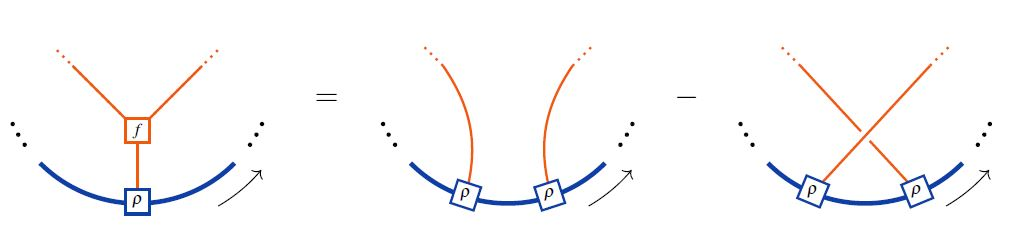# nLab Lie algebra object

Contents

### Context

#### Lie theory

Background

Smooth structure

Higher groupoids

Lie theory

∞-Lie groupoids

∞-Lie algebroids

Formal Lie groupoids

Cohomology

Homotopy

Related topics

Examples

$\infty$-Lie groupoids

$\infty$-Lie groups

$\infty$-Lie algebroids

$\infty$-Lie algebras

#### Categorical algebra

internalization and categorical algebra

universal algebra

categorical semantics

# Contents

## Idea

The notion of Lie algebra may be formulated internal to general linear monoidal categories (tensor categories). This general definition of Lie algebra objects internal to tensor categories subsumes variants of Lie algebras such as super Lie algebras.

## Definition

### As internal Lie algebras

Consider a commutative unital ring $k$, and a (strict for simplicity) symmetric monoidal $k$-linear category $(\mathcal{C},\otimes,1)$ with braiding $\tau$.

A Lie algebra object in $(\mathcal{C},\otimes,1,\tau)$ is

1. an object

$L \in \mathcal{C}$
2. morphism (the Lie bracket)

$[-,-] \;\colon\; L \otimes L \to L$

such that the following conditions hold:

1. $\left[-,\left[-,-\right]\right] + \left[-,\left[-,-\right]\right] \circ(id_L\otimes\tau_{L,L}) \circ(\tau\otimes id_L) + \left[-,\left[-,-\right]\right] \circ (\tau_{L,L}\otimes id_L)\circ (id_L\otimes\tau_{L,L}) = 0$
2. skew-symmetry:

\begin{aligned} & \phantom{+} [-,-] \\ & + [-,-]\circ \tau_{L,L} \\ & = \phantom{+} 0 \end{aligned}

Equivalently, in string diagram-notation in the ambient tensor category, the Lie action property looks as follows:\begin{aligned} \Leftrightarrow & \;\;\;\;\; \rho(f(x,y),z) \;=\; \rho(y,\rho(x,z)) - \rho(x,\rho(y,z)) \\ \underset{ {f = [-,-]} \atop {Lie\;bracket} }{ \Leftrightarrow } & \;\;\;\;\; \underset{ {Lie\;action\;property} }{ \underbrace{ \rho([x,y],z) \;=\; \rho(y,\rho(x,z)) - \rho(x,\rho(y,z)) } } \\ \underset{ {\rho = -[-,-]} \atop {adjoint\;action} }{ \Leftrightarrow } & \;\;\;\;\; \underset{ {Jacobi\;identity} }{ \underbrace{ [[x,y],z] \;=\; - [y,[x,z]] + [x,[y,z]] } } \end{aligned}

where the last line shows the equivalence to the Jacobi identity on the Lie algebra object itself in the case that the Lie action is the adjoint action.

graphics from Sati-Schreiber 19c

In the language of Jacobi diagrams this is called the STU-relation. and is the reason behind the existence of Lie algebra weight systems in knot theory. For more see also at metric Lie representation.

### As algebras over the Lie operad

Equivalently, Lie algebra objects are the algebras over an operad over a certain quadratic operad, called the Lie operad, which is the Koszul dual of the commutative algebra operad.

## Examples

Examples of types of Lie algebra objects:

(…)

### Examples

For references on super Lie algebras see there.

On Leibniz algebras as Lie algebra objects in suitable tensor categories of linear maps: